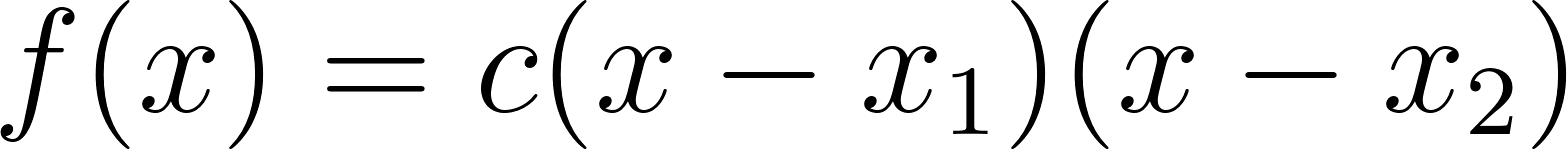×
Get Full Access to Calculus: Early Transcendentals - 1 Edition - Chapter 1.2 - Problem 63ae
Get Full Access to Calculus: Early Transcendentals - 1 Edition - Chapter 1.2 - Problem 63ae

×

# Parabola vertex property. Prove that if a parabola crossesISBN: 9780321570567 2

## Solution for problem 63AE Chapter 1.2

Calculus: Early Transcendentals | 1st Edition

• Textbook Solutions
• 2901 Step-by-step solutions solved by professors and subject experts
• Get 24/7 help from StudySoup virtual teaching assistantsCalculus: Early Transcendentals | 1st Edition

4 5 1 335 Reviews
24
0
Problem 63AE

Parabola vertex properties: Prove that if a parabola crosses the x-axis twice, the x-coordinate of the vertex of the parabola is halfway between the x-intercepts.

Step-by-Step Solution:

Step 1 of 3

The equation of parabola having zeros atandis,Step 2 of 3

Step 3 of 3

##### ISBN: 9780321570567

The full step-by-step solution to problem: 63AE from chapter: 1.2 was answered by , our top Calculus solution expert on 03/03/17, 03:45PM. This textbook survival guide was created for the textbook: Calculus: Early Transcendentals, edition: 1. This full solution covers the following key subjects: parabola, vertex, crosses, halfway, intercepts. This expansive textbook survival guide covers 85 chapters, and 5218 solutions. Calculus: Early Transcendentals was written by and is associated to the ISBN: 9780321570567. Since the solution to 63AE from 1.2 chapter was answered, more than 375 students have viewed the full step-by-step answer. The answer to “?Parabola vertex properties: Prove that if a parabola crosses the x-axis twice, the x-coordinate of the vertex of the parabola is halfway between the x-intercepts.” is broken down into a number of easy to follow steps, and 25 words.

Unlock Textbook Solution